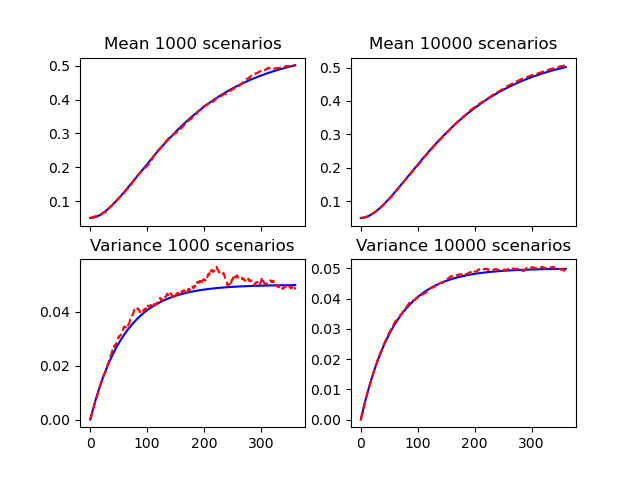# Short rate mean and variance#

The means and variances of the simulated short rates.

For each $$t_i$$, the mean and the variance of the short rate $$r(t_i)$$ should converge to $$E\{r(t_i) | \mathcal{F}_{0}\}$$ and $$Var\{r(t_i) | \mathcal{F}_{0}\}$$.import modelx as mx
import matplotlib.pyplot as plt

fig, axs = plt.subplots(2, 2, sharex=True, sharey=False)

for size, h in zip([1000, 10000], [0, 1]):
HW.scen_size = size
axs[0, h].plot(range(HW.step_size + 1), HW.E_rt(), "b-")
axs[0, h].plot(range(HW.step_size + 1), HW.mean_short_rate(), "r--")
axs[0, h].set_title('Mean ' + str(size) + ' scenarios')
axs[1, h].plot(range(HW.step_size + 1), HW.Var_rt(), "b-")
axs[1, h].plot(range(HW.step_size + 1), HW.var_short_rate(), "r--")
axs[1, h].set_title('Variance ' + str(size) + ' scenarios')


Total running time of the script: ( 0 minutes 0.395 seconds)

Gallery generated by Sphinx-Gallery